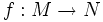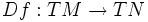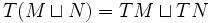# Tangent bundle functor

## Definition

The tangent bundle functor is a functor from the category of differential manifolds with smooth maps to the category of differential manifolds with smooth maps, defined as follows:

• On objects: It sends a differential manifold$M$ to its tangent bundle$TM$
• On morphisms: It sends a smooth map$f:M \to N$ of differential manifolds to its differential,$Df: TM \to TN$

The tangent bundle functor comes with a natural transformation to the identity functor: namely, the bundle map from$TM$ to$M$. There is also a natural transformation from the identity functor: namely, the zero section of$M$ in$TM$.

## Properties of the functor

### Product-preserving functor

This covariant functor is product-preserving: applying the functor to a product of objects in a category, is equivalent to taking the product after applying the functor
View other product-preserving functors

The tangent bundle functor preserves products; in other words, we have:$T(M \times N) \cong TM \times TN$

### Coproduct-preserving functor

This covariant functor commutes with coproducts: applying the functor after taking coproducts, has the same effect as taking the coproduct after applying the functor
View other coproduct-preserving functors

The tangent bundle functor preserves coproducts; in other words, we have:$T(M \sqcup N) = TM \sqcup TN$